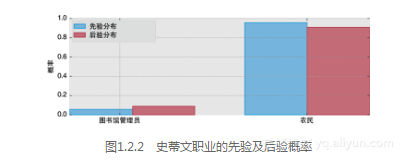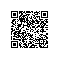# 《贝叶斯方法：概率编程与贝叶斯推断》一1.2　我们的贝叶斯框架

### 1.2　我们的贝叶斯框架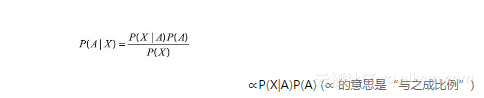### 1.2.1　不得不讲的实例：抛硬币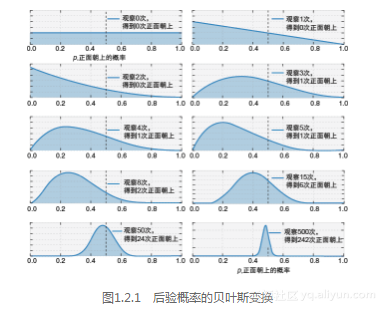### 1.2.2　实例：图书管理员还是农民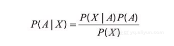P（X）可以解释为：任何人对史蒂文的描述和史蒂文邻居的描述一致的概率。现在这种形式有点难以理解，我们将其做一些逻辑上的改造：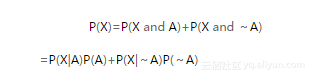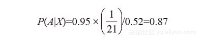%matplotlib inline
from IPython.core.pylabtools import figsize
import numpy as np
from matplotlib import pyplot as plt
figsize(12.5, 4)
plt.rcParams['savefig.dpi'] = 300
plt.rcParams['figure.dpi'] = 300

colors = ["#348ABD", "#A60628"]
prior = [1/21., 20/21.]
posterior = [0.087,1-0.087]
plt.bar([0, .7], prior, alpha=0.70, width=0.25,
color=colors, label="prior distribution",
lw="3", edgecolor="#348ABD")

plt.bar([0+0.25, .7+0.25], posterior, alpha=0.7,
width=0.25, color=colors,
label="posterior distribution",
lw="3", edgecolor="#A60628")

plt.xticks([0.20, 0.95], ["Librarian", "Farmer"])
plt.title("Prior and posterior probabilities of Steve's\
occupation")
plt.ylabel("Probability")
plt.legend(loc="upper left");